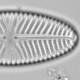## Uneven sampling of the gradient and segment-wise RMSEP

The root mean square error of prediction (RMSEP) is a measure of the performance of a transfer function for reconstructing past environmental conditions from fossil microfossil assemblages based on the modern relationship between species and the environment in a calibration set. If the RMSEP is large, reconstructions will be uncertain. It is calculated from the difference between the observed value of the environmental variable of interest at each site and the predictions made under cross-validation. The simplest cross-validation scheme is leave-one-out cross-validation where each observation is omitted in turn and its environmental value predicted from the remaining n-1 observations. Other cross-validation schemes are available, the differences are not important here.

Typically the RMSEP is given for the entire calibration set, but the uncertainty is not necessarily constant along the environmental variable of interest. Variability in the uncertainty could reflect the ecological sensitivity of the assemblages: perhaps at high values of the environmental variable the ecological response saturates and so only uncertain predictions are possible. Planktonic-foraminifera sea-surface temperate transfer functions give an example of this in cold water where the ecological response saturates with a mono-dominant N. pachyderma (sin) assemblage. Alternatively, changes in the uncertainty along the environmental gradient could reflect the sampling design, with low uncertainty in well-sampled parts of the gradient, and higher uncertainty in less well-sampled parts of the gradient. This issue was explored in Telford and Birks (2011). With weighted averaging based methods, taxa abundant in the well-sampled parts of the gradient have precise estimates of their optima and hence predictions are precise, and vice versa. With the modern analogue technique, observations from densely-sampled portions of the gradient have many suitable analogues, so are precisely predicted and vice versa. The more uneven the sampling is, the worse the problem, and the modern analogue technique is more sensitive to this problem than weighted averaging, which is more sensitive than maximum likelihood.

Since the RMSEP might not be constant along the environmental gradient, it can be useful to calculate the RMSEP for different portions of the gradient.

First load some data and the rioja package (these functions could easily be rewritten to use output from the analogue package).

```library(rioja)
data(SWAP)
spp<-SWAP\$spec
env<-SWAP\$pH
```

Now a function to calculate and plot segment-wise RMSEP.

```
segmentwise.rmse <- function(mod, ng = 10, k = 1, plot = TRUE, ...){
if(is.null(mod\$residuals.cv)){
if(class(mod) == "MAT"){
r <- mod\$fitted.values[,k]-mod\$x
perf<-performance(mod)\$object
}else{
stop("Need cross-validated model to calculate RMSEP")
}
}else{
r <- mod\$residuals.cv[,k]
perf<-performance(mod)\$crossval
}
breaks <- seq(min(mod\$x),max(mod\$x), length=ng)
envcut <- cut(mod\$x,breaks=breaks, include.lowest=TRUE)
segRMSEP <- tapply(r,envcut,function(x)sqrt(mean(x^2)))
allsegRMSEP <- sqrt(mean(segRMSEP^2))

if(plot){
hist(mod\$x, breaks=breaks, col="grey70", border=NA, ...)
par(new=T)
mid<-((c(breaks,NA)+c(NA,breaks))/2)
mid<-mid[!is.na(mid)]
plot(mid,segRMSEP, type="n", xlim=par()\$usr[1:2],xaxs="i", yaxt="n", ylab="", xlab="", col=2)
lines(breaks,c(segRMSEP,segRMSEP), type="S", col=3)
axis(4)
mtext("RMSEP", side=4, line=1.5)
abline(h = perf[k,"RMSE"], col=2, lty=2)
abline(h = allsegRMSEP, col=4, lty=2)
}
list(breaks = breaks, segRMSEP = segRMSEP, allsegRMSEP = allsegRMSEP)
}
```

The argument `ng` sets the number of segments to use. Ten is the default used to calculate maximum bias in rioja, so is used here as well. `k` sets the column of the model output to use. For example, with WA, the first column is for the inverse deshrinking model, and the second for the classical deshrinking model. Run `performance(mod)` to see which model is in which column. The code calculates the overall RMSEP based on the individual segments. I’m not sure how useful this number is, but as people have asked for it, I have included it.

Now we can use this function.

```modWA<-crossval(WA(spp, env))
modMAT<-MAT(spp,env)
x11(4.5,4.5); par(mar=c(3,3,1,3), mgp=c(1.5, 0.5, 0))
segmentwise.rmse(modWA, k=1, main="WA", xlab="pH")
segmentwise.rmse(modMAT, k=5, main="MAT", xlab="pH")
```Grey histogram showing distribution of observations along the pH gradient. Green line shows segment-wise RMSEP for a WA model, red dashed line shows the model RSMEP, and the blue dashed line shows the RMSEP for the model calculated from the segment-wise RMSEPs.

It is not necessarily bad that the RSMEP is not constant along the environmental gradient. If we are concerned with predictions from the one end of the gradient, weak performance at the other end need not concern us, indeed the RMSEP in the section we are interested in may be lower than that reported for the whole calibration set.

I’ll include this function in the next version of palaeoSig.1.Nina Desianti says: